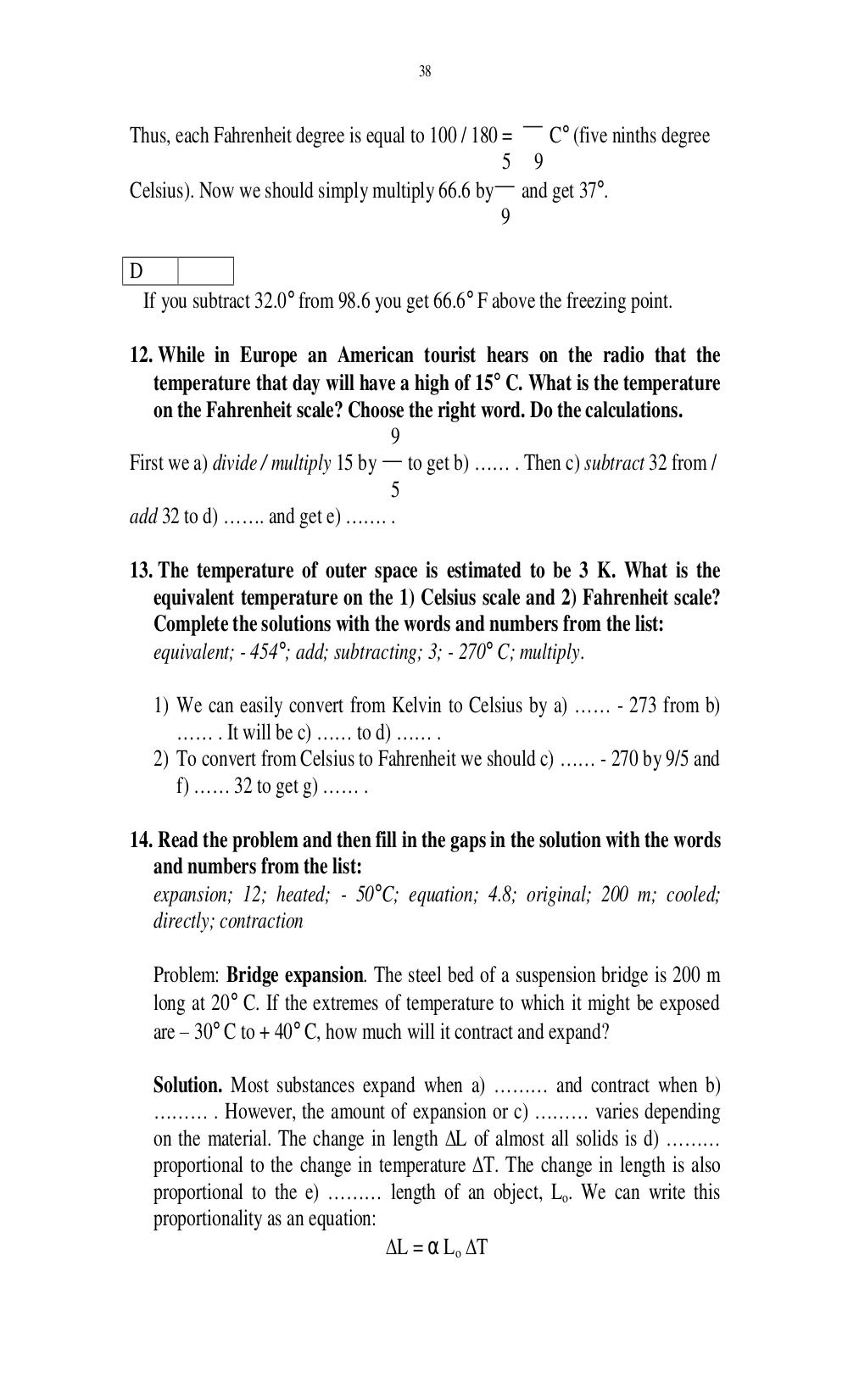# Английский язык. Ильичева Н.А - 38 стр.

Составители:

Рубрика:

• ## Иностранный язык38
Thus, each Fahrenheit degree is equal to 100 / 180 = C° (five ninths degree
5 9
Celsius). Now we should simply multiply 66.6 by and get 37°.
9
D
If you subtract 32.0° from 98.6 you get 66.6° F above the freezing point.
12. While in Europe an American tourist hears on the radio that the
temperature that day will have a high of 15° C. What is the temperature
on the Fahrenheit scale? Choose the right word. Do the calculations.
9
First we a) divide / multiply 15 by to get b) …… . Then c) subtract 32 from /
5
add 32 to d) ……. and get e) ……. .
13. The temperature of outer space is estimated to be 3 K. What is the
equivalent temperature on the 1) Celsius scale and 2) Fahrenheit scale?
Complete the solutions with the words and numbers from the list:
equivalent; - 454°; add; subtracting; 3; - 270° C; multiply.
1) We can easily convert from Kelvin to Celsius by a) …… - 273 from b)
…… . It will be c) …… to d) …… .
2) To convert from Celsius to Fahrenheit we should c) …… - 270 by 9/5 and
f) …… 32 to get g) …… .
14. Read the problem and then fill in the gaps in the solution with the words
and numbers from the list:
expansion; 12; heated; - 50°C; equation; 4.8; original; 200 m; cooled;
directly; contraction
Problem: Bridge expansion. The steel bed of a suspension bridge is 200 m
long at 20° C. If the extremes of temperature to which it might be exposed
are 30° C to + 40° C, how much will it contract and expand?
Solution. Most substances expand when a) ……… and contract when b)
……… . However, the amount of expansion or c) ……… varies depending
on the material. The change in length L of almost all solids is d) ………
proportional to the change in temperature T. The change in length is also
proportional to the e) ……… length of an object, L
o
. We can write this
proportionality as an equation:
L = α L
o
T38

Thus, each Fahrenheit degree is equal to 100 / 180 =   C° (five ninths degree
5 9
Celsius). Now we should simply multiply 66.6 by and get 37°.
9

D
If you subtract 32.0° from 98.6 you get 66.6° F above the freezing point.

12. While in Europe an American tourist hears on the radio that the
temperature that day will have a high of 15° C. What is the temperature
on the Fahrenheit scale? Choose the right word. Do the calculations.
9
First we a) divide / multiply 15 by   to get b) …… . Then c) subtract 32 from /
5
add 32 to d) ……. and get e) ……. .

13. The temperature of outer space is estimated to be 3 K. What is the
equivalent temperature on the 1) Celsius scale and 2) Fahrenheit scale?
Complete the solutions with the words and numbers from the list:
equivalent; - 454°; add; subtracting; 3; - 270° C; multiply.

1) We can easily convert from Kelvin to Celsius by a) …… - 273 from b)
…… . It will be c) …… to d) …… .
2) To convert from Celsius to Fahrenheit we should c) …… - 270 by 9/5 and
f) …… 32 to get g) …… .

14. Read the problem and then fill in the gaps in the solution with the words
and numbers from the list:
expansion; 12; heated; - 50°C; equation; 4.8; original; 200 m; cooled;
directly; contraction

Problem: Bridge expansion. The steel bed of a suspension bridge is 200 m
long at 20° C. If the extremes of temperature to which it might be exposed
are – 30° C to + 40° C, how much will it contract and expand?

Solution. Most substances expand when a) ……… and contract when b)
……… . However, the amount of expansion or c) ……… varies depending
on the material. The change in length ∆L of almost all solids is d) ………
proportional to the change in temperature ∆T. The change in length is also
proportional to the e) ……… length of an object, Lo. We can write this
proportionality as an equation:
∆L = α Lo ∆T